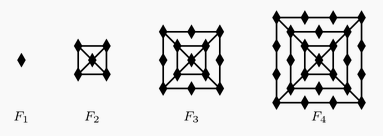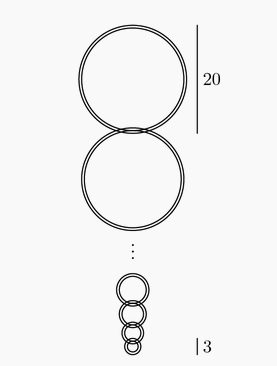###### back to index | new

The figures $F_1$, $F_2$, $F_3$, and $F_4$ shown are the first in a sequence of figures. For $n\ge3$, $F_n$ is constructed from $F_{n - 1}$ by surrounding it with a square and placing one more diamond on each side of the new square than $F_{n - 1}$ had on each side of its outside square. For example, figure $F_3$ has $13$ diamonds. How many diamonds are there in figure $F_{20}$?Let $a + ar_1 + ar_1^2 + ar_1^3 + \cdots$ and $a + ar_2 + ar_2^2 + ar_2^3 + \cdots$ be two different infinite geometric series of positive numbers with the same first term. The sum of the first series is $r_1$, and the sum of the second series is $r_2$. What is $r_1 + r_2$?

The tower function of twos is defined recursively as follows: $T(1) = 2$ and $T(n + 1) = 2^{T(n)}$ for $n\ge1$. Let $A = (T(2009))^{T(2009)}$ and $B = (T(2009))^A$. What is the largest integer $k$ such that $\underbrace{\log_2\log_2\log_2\ldots\log_2B}_{k\text{ times}}$ is defined?

The first two terms of a sequence are $a_1 = 1$ and $a_2 = \frac {1}{\sqrt3}$. For $n\ge1$, $a_{n + 2} = \frac {a_n + a_{n + 1}}{1 - a_na_{n + 1}}.$ What is $|a_{2009}|$?

The fifth and eighth terms of a geometric sequence of real numbers are $7!$ and $8!$ respectively. What is the first term?

Let $a_1,a_2,\ldots$ be a sequence determined by the rule $a_n=a_{n-1}/2$ if $a_{n-1}$ is even and $a_n=3a_{n-1}+1$ if $a_{n-1}$ is odd. For how many positive integers $a_1 \le 2008$ is it true that $a_1$ is less than each of $a_2$, $a_3$, and $a_4$?

A sequence $(a_1,b_1)$, $(a_2,b_2)$, $(a_3,b_3)$, $\ldots$ of points in the coordinate plane satisfies $(a_{n + 1}, b_{n + 1}) = (\sqrt {3}a_n - b_n, \sqrt {3}b_n + a_n)$ for $n = 1,2,3,\ldots$. Suppose that $(a_{100},b_{100}) = (2,4)$. What is $a_1 + b_1$?

For each positive integer $n$, the mean of the first $n$ terms of a sequence is $n$. What is the $2008$th term of the sequence?

Let $A_0=(0,0)$. Distinct points $A_1,A_2,\dots$ lie on the $x$-axis, and distinct points $B_1,B_2,\dots$ lie on the graph of $y=\sqrt{x}$. For every positive integer $n,\ A_{n-1}B_nA_n$ is an equilateral triangle. What is the least $n$ for which the length $A_0A_n\geq100$?

Let $a, b, c, d$, and $e$ be five consecutive terms in an arithmetic sequence, and suppose that $a+b+c+d+e=30$. Which of $a, b, c, d,$ or $e$ can be found?

The geometric series $a+ar+ar^2\ldots$ has a sum of $7$, and the terms involving odd powers of $r$ have a sum of $3$. What is $a+r$?

A number of linked rings, each 1 cm thick, are hanging on a peg. The top ring has an outside diameter of 20 cm. The outside diameter of each of the outer rings is 1 cm less than that of the ring above it. The bottom ring has an outside diameter of 3 cm. What is the distance, in cm, from the top of the top ring to the bottom of the bottom ring?Given a finite sequence $S=(a_1,a_2,\ldots ,a_n)$ of $n$ real numbers, let $A(S)$ be the sequence $\left(\frac{a_1+a_2}{2},\frac{a_2+a_3}{2},\ldots ,\frac{a_{n-1}+a_n}{2}\right)$ of $n-1$ real numbers. Define $A^1(S)=A(S)$ and, for each integer $m$, $2\le m\le n-1$, define $A^m(S)=A(A^{m-1}(S))$. Suppose $x>0$, and let $S=(1,x,x^2,\ldots ,x^{100})$. If $A^{100}(S)=(1\/2^{50})$, then what is $x$?

A sequence $a_1,a_2,\dots$ of non-negative integers is defined by the rule $a_{n+2}=|a_{n+1}-a_n|$ for $n\geq 1$. If $a_1=999$, $a_2<999$ and $a_{2006}=1$, how many different values of $a_2$ are possible?

Big Al, the ape, ate 100 bananas from May 1 through May 5. Each day he ate six more bananas than on the previous day. How many bananas did Big Al eat on May 5?

A sequence of numbers starts with $1$, $2$, and $3$. The fourth number of the sequence is the sum of the previous three numbers in the sequence: $1+2+3=6$. In the same way, every number after the fourth is the sum of the previous three numbers. What is the eighth number in the sequence?

If the sum of an arithmetic progression of six positive integer terms is 78, what is the greatest possible difference between consecutive terms?

For a particular sequence $a_1 = 3$, $a_2 = 5$ and $a_n = a_{n -1} - a_{n -2}$, for $n \ge 3$. What is the $2015^{th}$ term in this sequence?

When $\frac{1}{98}$ is expressed as a decimal, what is the $10^{th}$ digit to the right of the decimal point?

The Incredible Hulk can double the distance he jumps with each succeeding jump. If his first jump is 1 meter, the second jump is 2 meters, the third jump is 4 meters, and so on, then on which jump will he first be able to jump more than 1 kilometer?

The sum of six consecutive positive integers is 2013. What is the largest of these six integers?

How many terms are in the arithmetic sequence $13$, $16$, $19$, $\dotsc$, $70$, $73$?

Five positive consecutive integers starting with $a$ have average $b$. What is the average of $5$ consecutive integers that start with $b$?

A sequence of natural numbers is constructed by listing the first $4$, then skipping one, listing the next $5$, skipping $2$, listing $6$, skipping $3$, and, on the $n$th iteration, listing $n+3$ and skipping $n$. The sequence begins $1,2,3,4,6,7,8,9,10,13$. What is the $500,\!000$th number in the sequence?

When counting from $3$ to $201$, $53$ is the $51^{st}$ number counted. When counting backwards from $201$ to $3$, $53$ is the $n^{th}$ number counted. What is $n$?